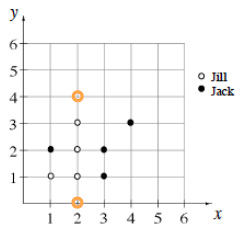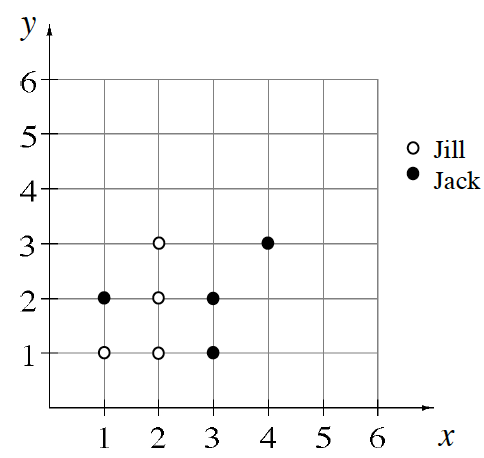### Home > CC1 > Chapter 5 > Lesson 5.3.1 > Problem5-73

5-73.

Jack and Jill were each placing points on the grid shown below. Jack’s points are the full circles, and Jill’s are the open circles. Homework Help ✎

1. Record Jack and Jill’s points as ordered pairs.

Remember, in an ordered pair, the x-coordinate (showing the placement along the x-axis) is listed first and the
y-coordinate (showing the placement along the y-axis) is listed second, as in (x, y).

Jill's ordered pairs are (1, 1), (2, 1), (2, 2), and (2, 3).
Can you name Jack's ordered pairs?

2. Give the coordinates of one more point that Jill could draw so that she has four of her points in a row.

Can you spot the possible points? Here's a clue: the point should be at the 2 value on the x-axis, so the ordered pair will look something like this (2, y).

The two possible points are now plotted. Can you record their ordered pairs?